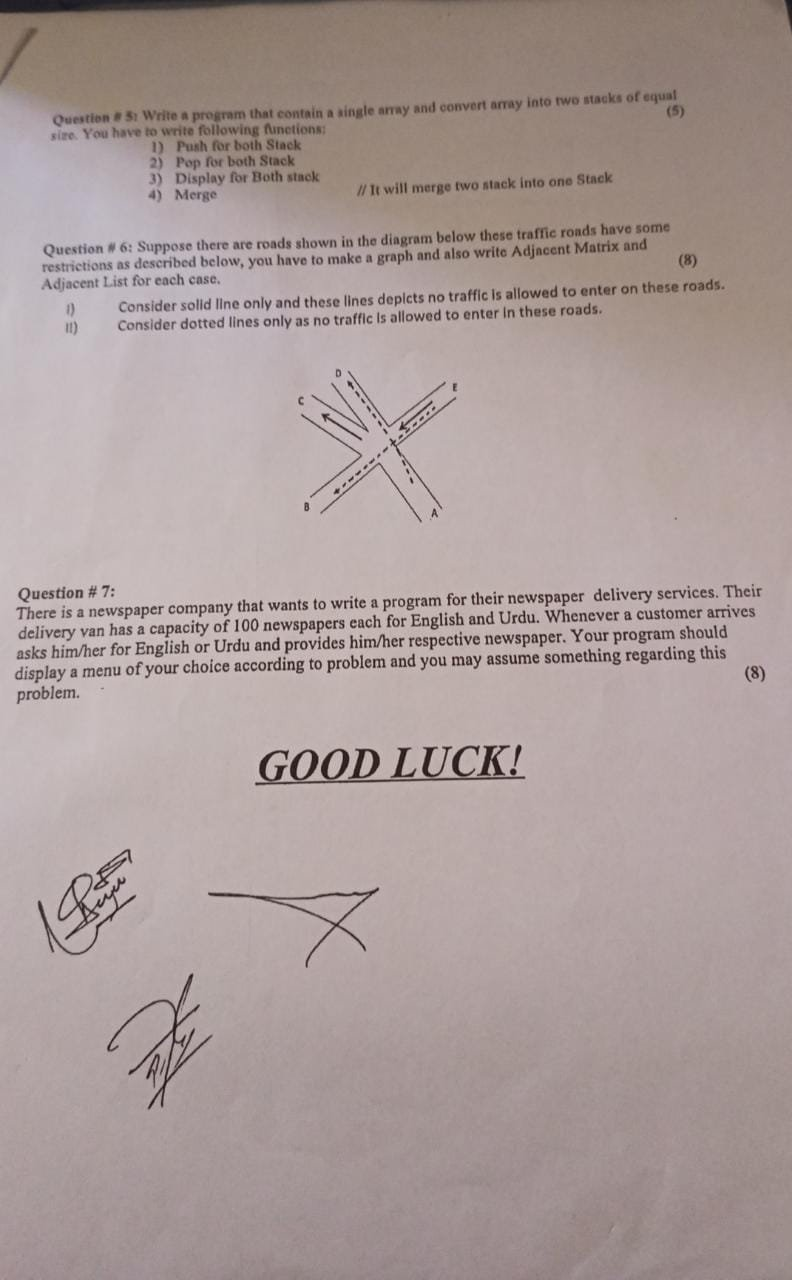# Data structure and algorithm all previous/ past question papers

-Data structure and algorithm –Computer Science all subject past paper  Data structure and algorithm- Data structure and algorithm Project idea -Data structure and algorithm MCQ -Data structure and algorithm Interview Question  –Computer Science all courses -Technology –University Past Paper -Programming language –Question paperold paper

## Data structure and algorithm Final in 2021### Mid term Exam Data structure and algorithm paper in 2021

Data structure and algorithm

Q.NO.1

Write a program to convert an infix expression that includes (,), +,-,*and / to postfix and apply it on the following expressions.

1. p+q*r+(s*t)
2. a*(b+c)/d

Q.No.2

What do you know about stack? Describe the purpose of each of the following stack operations

1. Push (), Pop (), Peek (), Isfull () and Isempty ()
2. Convert a*(b*c) + (d*e) into its postfix form using stack operations

Q.No.3

(A)

Let’s suppose if a queue is filled at a Rear end and there is an empty space at Front end then how can one insert new element into the queue? What do you know about queuing theory? How priority queue is better than double ended queue?

(B)

How link list is better than array? Compare the pros and cons of array and link list.

GOOD LUCK

## Data structure and algorithm Sessional I in 2020

DSA S1

Data structure and algorithm Sessional II in 2020

DSA S2

DSA Final

## Data structure and algorithm Sessional II in 2019

Q1: Write a program to convert an infix expression that includes (.)- *and la positia on the following expressions.

P+q*r+(s*t)

a*(b+c)/d

Q2: What do you know about stack? Describe the purpose of each of the following stack operations (I) Push () (II) Pop () (III) Peek () (iv) Isfull ( ) (v) Isempty () (in Convert a* (b*e) + (d*e) into its postfix form using stack operations.

Q3:Lets suppose if a queue is tilled at Rear end and there is an empty space at Front end then how can one insert new element into the queue? What do you know about queuing theory? How priority queue ? better than double ended queue?

Q3:How Tint list is better than Array? Compare the pros and cons of array and link list.

#Data structure and algorithm #Computer Science all subject past paper  #Data structure and algorithm- #Data structure and algorithm Project idea #Data structure and algorithm MCQ #Data structure and algorithm Interview Question  #Computer Science all courses #Technology #University Past Paper #Programming language #Question paper #old paper

Scroll to Top